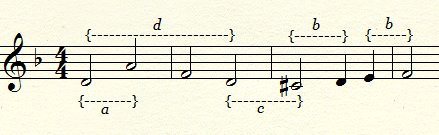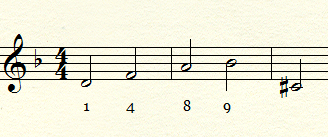Alphametics is the art of substituting letters for numbers in a valid simple addition sum. The letters must correspond to a particular word. The first modern alphametic was published by H.E. Dudeney in the July 1924 issue of Strand Magazine:

SEND +
MORE
-----
MONEY

Only one solution is valid:

9567 +
1085
-----
10652

There are some interesting and compelling alphametics corresponding to Bach and more specifically to his magnificent Art of Fugue and A Musical Offering.

First of all, let us consider two alphametics regarding BACH AND MUSIC:

BACH +
AND
-----

MUSIC

This yields two results:

9738 +
725
-----
10463

9735 +
728
-----
10463

Bach was very interested in numerology and considered the number five to be his personal number. The sum of the results (in red) of the above alphametic is five:

1+4+6+3=14

14 can be 'reduced' to five by simple addition:

1+4=5

The sum of the letters in Bach's name also result in 5:

BACH = 14 (2+1+3+8=14)
J.S.BACH = 14

Next, let us consider some Alphametics around DIE KUNST DER FUGUE:

DIE (2) +
KUNST (4)
-----

FUGUE

804 +
12679 (Reduce to 7 - 1+2+6+7+9=25: 2+5=7)
-----
52324 (Reduce to 7 - 5+2+3+2+4=16: 1+6=7)

825 +
19495
-----
79630 (Reduce to 7 - 7+9+6+3=25: 2+5=7)

Now in French; L'ART DE LA FUGUE:

LART    +
DE (4)+
LA
-----
FUGUE

9847
65
98
-----
10205

9754 +
86 +
97
-----
10206

Now, take the sum of the results from DIE KUNST DER FUGUE and L'ART DE LA FUGUE:

52324 +
79630
------
131954

10205 +
10206
------
20411

131954 +
20411
------
152365

The sums of the individual numbers in the above correspond to successive Fibonacci Numbers:

1+3+1+9+5+4=23 (5)
2+0+4+1+1=8    (8)
1+5+2=8        (8)
1+5+2+3=11    (11)
6+5=11        (11)

Most interesting is how the final sum (152365) corresponds to the notes of the main subject in The Art of Fugue:a = Perfect 5th

b= Rising minor 2nd

c = falling Minor 2nd

d = Minor 3rd

Bach and the New Fibonacci Gematria

The first 25 numbers in the Fibonacci Sequence are:

0, 1, 1, 2, 3, 5, 8, 13, 21, 34, 55, 89, 144, 233, 377, 610, 987, 1597, 2584, 4181, 6765, 10946, 17711, 28657, 46368

Disregard the first zero and reduce the double digit numbers (i.e. 13 is 1+3=4, 21 is 2+1=3):

1,1,2,3,5,8,4,3,7,1,8,9,8,8,7,6,4,1,5,6,2,8,1,9

Now place these numbers in a 6 by 4 table:

1 1 2 3 5 8
4 3 7 1 8 9
8 8 7 6 4 1
5 6 2 8 1 9

Substitute the numbers for letters in the following manner to yield the following Gematria:

A E I/J N  R  W
B F  K  O  S  Y
C G  L  P  T  Y
D H  M  Q U/V Z

The first column corresponds to the Tonic, Subdominant and Dominant

1             4th                8th           5th
Tonic  Subdominant  Octave  Dominant

The letters also correspond to Pitch Classes resulting in the major/minor triad:

1            4                 8                       5
Root  Minor 3rd  Perfect 5th  Major 3rd

This corresponds to the main subject in The Art of Fugue:

D  A  F  D

1  8  4  1

In this Gematria, F is equivalent to 4 (minor 3rd) and 5 corresponds to F#, the sharpened third (Tierce de Picardie) used in the final note of a Minor key Perfect Cadence.

An interesting result is obtained if we intertwine the letters of BACH and W.T.C (Well Tempered Clavier):

1 6 --|

|-- 4 8 --| BACH

W.T.C |-- 8 4 --|

6 1 --| BACH

Now, consider the table with special reference to the bold, red digits:

1 1 2 3 5 8
4 3 7 1 8 9
8 8 7 6 4 1
5 6 2 8 1 9

The numbers correspond to the first four notes from the Royal Theme in A Musical Offering:

D  F  A  Bb

1  4  8   9Finally, there are interesting corrolations to Bach's name in the top row of the table:

J   (n)     S  (e   b   n)    B   A   C   H

10 14     19  5   2   14   2    1   3    8

1     5        1               5

This free website was made using Yola.

No HTML skills required. Build your website in minutes.